# Recipes with Fractions

Recipes with fractions are great for kids learning this important maths skill. Cooking helps teach kids practical applications for maths, and will help them attain fluency with fractions. Not only that, but cooking is fun! You and your child can mix things up in the kitchen with this fun fraction series. practise pairing down recipes, using real recipes from our Activities section of the site.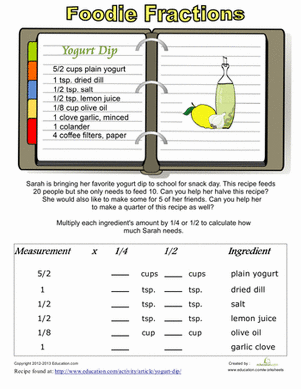### Recipe Conversion

Introduce your little maths champ to fraction multiplication in the kitchen! He'll get a taste of measurements using a recipe to calculate the fractions.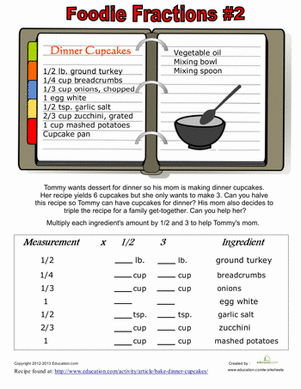### Recipe Fractions #2

Use food and maths together to learn fractions! Help your child multiply fractions to convert these recipes to smaller or larger portions.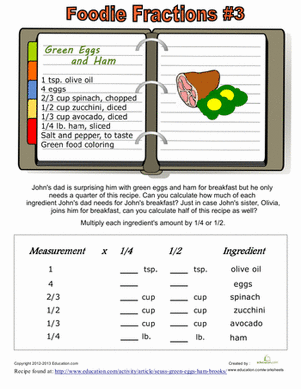### Recipe Fractions #3

Combine maths with the fun of cooking! practise fraction multiplication using the measurements of ingredients from a recipe.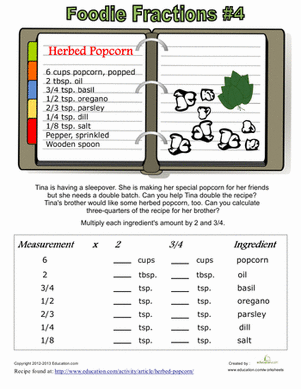### Recipe Fractions #4

Practise multiplying fractions while learning to cook in the kitchen! Your little mathematical chef will adjust the measurements of a real recipe.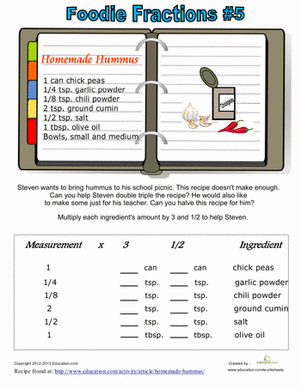### Recipe Fractions #5

Use food to make maths fun! practise multiplying fractions using a recipe. Your little maths whiz will adjust the recipe to make more and to make less.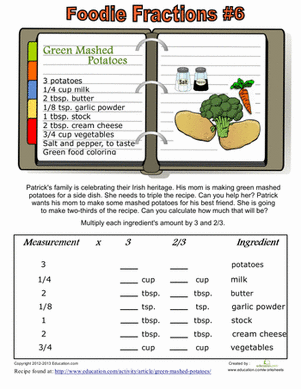### Recipe Fractions #6

Fraction practise can be fun when you add food into the mix! Your child will multiply fractions to change the amounts of ingredients in a recipe.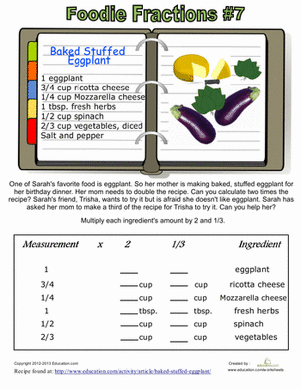### Recipe Fractions #7

Practise multiplying fractions using a real recipe. Your child will have a chance to learn about food as she learns about maths.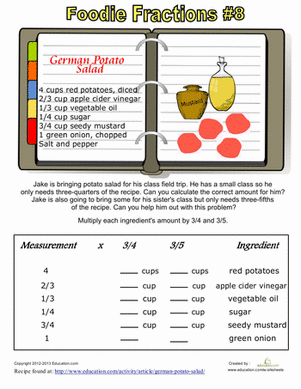### Recipe Fractions #8

Have some fun with fractions in the kitchen! Your little mathematician can be a little chef too, as he practices multiplying ingredient amounts with fractions.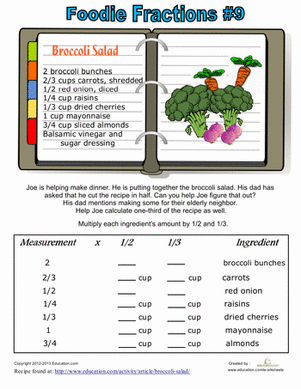### Recipe Fractions #9

Mix maths up by combining cooking and fractions! Your little star will practise multiplying fractions that are part of a recipe.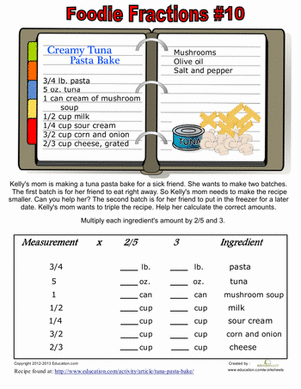### Recipe Fractions #10

Stir up a fun maths activity with foodie fraction multiplication! Use a real recipe to practise multiplying fractions.

Create new collection

0

### New Collection>

0Items

What could we do to improve Education.com?